The same current i = 2A is flowing in a wireframe as shown in the figure. The frame is a combination of two equilateral triangles ACD and CDE of side 1m. It is placed in uniform magnetic field B = 4T acting perpendicular to the plane of the frame. The magnitude of the magnetic force acting on the frame is: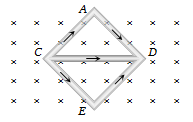1. 24 N                                         2. Zero

3. 16 N                                         4. 8 N

Concept Questions :-

Lorentz force
High Yielding Test Series + Question Bank - NEET 2020

Difficulty Level:

In the given figure net magnetic field at O will be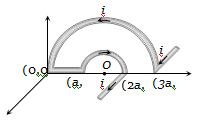(a) $\frac{2{\mu }_{0}i}{3\mathrm{\pi a}}\sqrt{4-{x}^{2}}$                                 (b) $\frac{{\mu }_{0}i}{3\mathrm{\pi a}}\sqrt{4+{x}^{2}}$

(c) $\frac{2{\mu }_{0}i}{3\mathrm{\pi a}}\sqrt{4+{x}^{2}}$                                  (d) $\frac{2{\mu }_{0}i}{3\mathrm{\pi a}}\sqrt{\left(4-{\mathrm{\pi }}^{2}\right)}$

Concept Questions :-

Magnetic field due to various cases
High Yielding Test Series + Question Bank - NEET 2020

Difficulty Level:

In the following figure a wire bent in the form of a regular polygon of n sides is inscribed in a circle of radius a. Net magnetic field at centre will be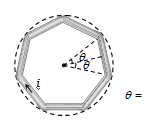(a) $\frac{{\mu }_{o}i}{2\mathrm{\pi a}}\mathrm{tan}\frac{\mathrm{\pi }}{n}$                                                (b) $\frac{{\mu }_{0}ni}{2\mathrm{\pi a}}\mathrm{tan}\frac{\mathrm{\pi }}{n}$

(c)$\frac{2}{\mathrm{\pi }}\frac{ni}{a}{\mu }_{0}\mathrm{tan}\frac{\mathrm{\pi }}{n}$                                             (d) $\frac{ni}{2a}{\mu }_{0}\mathrm{tan}\frac{\mathrm{\pi }}{n}$

Concept Questions :-

Magnetic field due to various cases
High Yielding Test Series + Question Bank - NEET 2020

Difficulty Level:

The unit vectors  are as shown below. What will be the magnetic field at O in the following figure?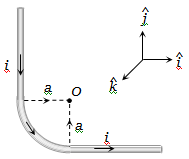(a) $\frac{{\mu }_{0}}{4\mathrm{\pi }}\frac{i}{a}\left(2-\frac{\mathrm{\pi }}{2}\right)\stackrel{^}{j}$             (b) $\frac{{\mu }_{0}}{4\mathrm{\pi }}\frac{i}{a}\left(2+\frac{\mathrm{\pi }}{2}\right)\stackrel{^}{j}$

(c) $\frac{{\mu }_{0}}{4\mathrm{\pi }}\frac{i}{a}\left(2+\frac{\mathrm{\pi }}{2}\right)\stackrel{^}{i}$              (d) $\frac{{\mu }_{0}}{4\mathrm{\pi }}\frac{i}{a}\left(2+\frac{\mathrm{\pi }}{2}\right)\stackrel{^}{k}$

Concept Questions :-

Magnetic field due to various cases
High Yielding Test Series + Question Bank - NEET 2020

Difficulty Level:

A particle of charge q and mass m moves in a circular orbit of radius r with angular speed $\omega$ . The ratio of the magnitude of its magnetic moment to that of its angular momentum depends on

(a)                         (b)

(c)                         (d)

Concept Questions :-

Magnetic moment
High Yielding Test Series + Question Bank - NEET 2020

Difficulty Level:

An elastic circular wire of length L carries a current I. It is placed in a uniform magnetic field $\stackrel{\to }{B}$ (out of paper) such that its plane is perpendicular to the direction of $\stackrel{\to }{B}$ . The wire will experience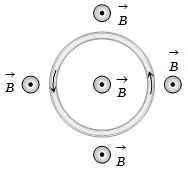(a) No force                            (b) A stretching force

(c) A compressive force            (d) A torque

Concept Questions :-

Current carrying loop : Force and torque
High Yielding Test Series + Question Bank - NEET 2020

Difficulty Level:

Wires 1 and 2 carrying currents ${i}_{1}$ and ${i}_{2}$ respectively are inclined at an angle $\theta$ to each other. What is the force on a small element dl of wire 2 at a distance of r from wire 1 (as shown in figure) due to the magnetic field of wire 1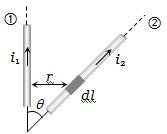(a)                               (b)

(c)                                (d)

Concept Questions :-

Force between current carrying wires
High Yielding Test Series + Question Bank - NEET 2020

Difficulty Level:

A conducting loop carrying a current I is placed in a uniform magnetic field pointing into the plane of the paper as shown. The loop will have a tendency to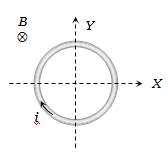(a) Contract                                        (b) Expand

(c) Move towards +ve x -axis               (d) Move towards -ve x -axis

Concept Questions :-

Current carrying loop : Force and torque
High Yielding Test Series + Question Bank - NEET 2020

Difficulty Level:

A current-carrying loop is placed in a uniform magnetic field in four different orientations, I, II, III & IV. The decreasing order of potential energy is :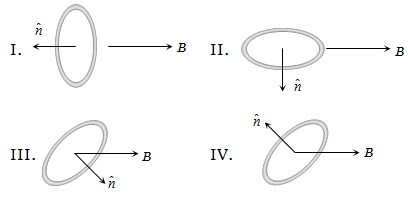(a) I > III > II > IV                               (b) I > II >III > IV
(c) I > IV > II > III                               (d) III > IV > I > II

Concept Questions :-

Current carrying loop : Force and torque
High Yielding Test Series + Question Bank - NEET 2020

Difficulty Level:

A metallic block carrying current I is subjected to a uniform magnetic induction $\stackrel{⇁}{B}$ as shown in the figure. The moving charges experience a force given $\stackrel{⇁}{F}$ by ........... which results in the lowering of the potential of the face ........ Assume the speed of the carriers to be v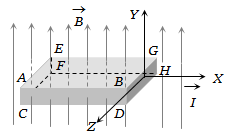(a) $eVB\stackrel{^}{k}$ , ABCD                                (b) $eVB\stackrel{^}{k}$ , EFGH

(c) $-eVB\stackrel{^}{k}$ , ABCD                              (d) $-eVB\stackrel{^}{k}$ , EFGH

Concept Questions :-

Lorentz force